# Wilcox Solar Observatory Magnetograms

## #8030 - 8039, 2004.07.29 - 2004.08.05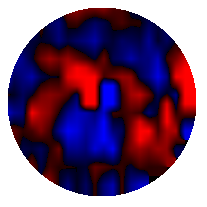```WSO Magnetogram sn=8030	 observed at 2004:07:29_22h:16m:26s
Central Meridian Carrington Longitude CT2019:260
Sky = 0 (5 worst)
trouble=00004000 NOTE:
12 intensity variance exceeds 5.0
```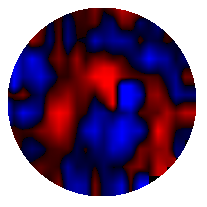```WSO Magnetogram sn=8031	 observed at 2004:07:31_00h:42m:07s
Central Meridian Carrington Longitude CT2019:245
Sky = 0 (5 worst)
trouble=00004000 NOTE:
12 intensity variance exceeds 5.0
```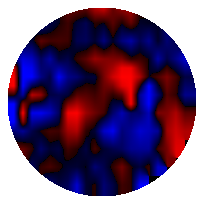```WSO Magnetogram sn=8032	 observed at 2004:07:31_22h:54m:48s
Central Meridian Carrington Longitude CT2019:233
Sky = 0 (5 worst)
trouble=00004000 NOTE:
12 intensity variance exceeds 5.0
```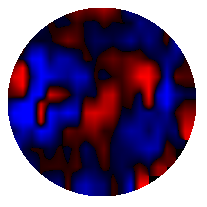```WSO Magnetogram sn=8033	 observed at 2004:08:02_00h:46m:17s
Central Meridian Carrington Longitude CT2019:219
Sky = 0 (5 worst)
trouble=00004000 NOTE:
12 intensity variance exceeds 5.0
```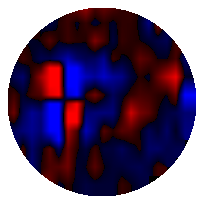```WSO Magnetogram sn=8034	 observed at 2004:08:03_20h:24m:01s
Central Meridian Carrington Longitude CT2019:195
Sky = 0 (5 worst)
trouble=00004000 NOTE:
12 intensity variance exceeds 5.0
```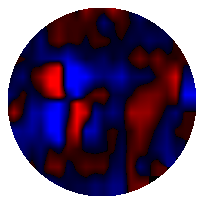```WSO Magnetogram sn=8035	 observed at 2004:08:03_22h:32m:10s
Central Meridian Carrington Longitude CT2019:194
Sky = 0 (5 worst)
trouble=00004000 NOTE:
12 intensity variance exceeds 5.0
```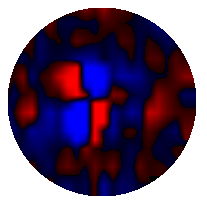```WSO Magnetogram sn=8036	 observed at 2004:08:04_20h:48m:51s
Central Meridian Carrington Longitude CT2019:181
Sky = 0 (5 worst)
trouble=00004000 NOTE:
12 intensity variance exceeds 5.0
```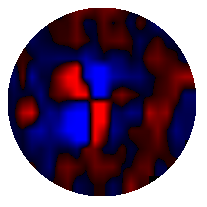```WSO Magnetogram sn=8037	 observed at 2004:08:04_22h:56m:32s
Central Meridian Carrington Longitude CT2019:180
Sky = 0 (5 worst)
trouble=00004000 NOTE:
12 intensity variance exceeds 5.0
```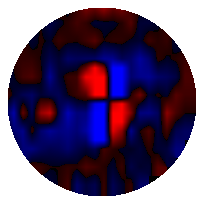```WSO Magnetogram sn=8038	 observed at 2004:08:05_21h:36m:39s
Central Meridian Carrington Longitude CT2019:168
Sky = 0 (5 worst)
trouble=00004000 NOTE:
12 intensity variance exceeds 5.0
```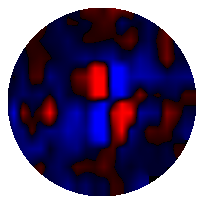```WSO Magnetogram sn=8039	 observed at 2004:08:05_23h:44m:23s
Central Meridian Carrington Longitude CT2019:166
Sky = 0 (5 worst)
trouble=00004000 NOTE:
12 intensity variance exceeds 5.0
```